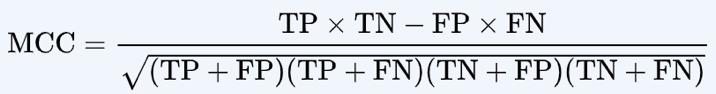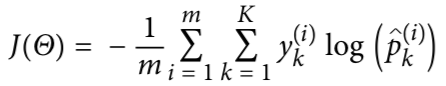# Evaluation metrics for classification

### Accuracy/Error rate

ACC = (TP+TN)/(P+N)

ERR = (FP+FN)/(P+N) = 1-ACC

### Precision/Recall/F1

Precision = TP/(TP+FP)-- positive predictive value

Recall= TP/(TP+FN)  -- true positive rate

F1=1/(1/precision+1/recall)

### ROC

True positive rate (TPR): the ratio of positive instances that are correctly classified as positive

TPR = TP/(TP+FN) = recall

True negative rate (TNR): the ratio of negative instances that are correctly classified as negative

TNR = TN/(TN+FP) = specify

False positive rate (FPR): the ratio of negative instances that are incorrectly classified as positive.

FPR = FN/(TN+FP) = 1-specify

ROC: TPR vs FPR

### Matthews correlation coefficient### Logarithm loss/cross entropyposted @ 2019-11-07 23:38  Sherrrry  阅读(134)  评论(0编辑  收藏  举报